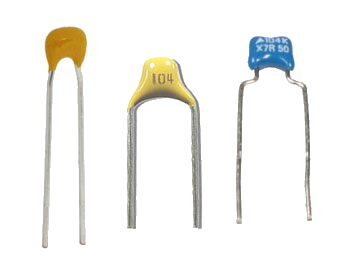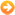CART ARTICLES 0 TOTAL 0.00€
You are in CART
Shopping details
You are in Home | Products | Capacitors | MKT Multilayer CapacitorsCapacitorsMKT Multilayer Capacitors

The acronym MLCC comes from "Multilayer Ceramic Capacitor", this is "Multilayer Ceramic Capacitor". Basically, these multilayer ceramic capacitors consist of a large number of individual capacitors stacked on top of each other, connected in parallel. But first before we have to understand that it is a ceramic capacitor.

The Ceramic Capacitor is a passive electronic component that is capable of storing an electrical charge, it behaves like a filter that blocks direct current and allows alternating current to flow without any problem.

The capacity of a condenser varies with temperature. The different dielectrics of the many types of capacitors show large differences in temperature dependence. Based on these differences, we classify ceramic capacitors into Class 1, 2 and 3.

• Class 1 ceramics: Capacitors offer high stability and low losses for resonant circuit applications. Ex: NP0, C0G, P100, N75, N100,… N1500. In NP0 (NPO sometimes so called in Europe) the temperature characteristic is approximately 0. But there are also those with negative temperature coefficients like all N100, N75, etc. For example, an N1500 will have a deviation of −1500 ppm / ºK. Often times these with negative temperature coefficients are used to compensate for the effects of temperature in a circuit, where there are other components with positive temperature coefficients. P100 would be a class 1 capacitor where the deviation is +100 ppm / ºK
• Class 2 ceramics - Offer high volume efficiency for damping, bypass and coupling applications. Ex: X5R, X7R. It used to be called a low-frequency ceramic capacitor (Low-frequency ceramic capacitor), which refers to the ferroelectric ceramic as the dielectric capacitor, so it is also called a ferroelectric ceramic capacitor. This type of capacitor has a large specific capacity, a non-linear change in capacity with temperature, and a large loss.
• Class 3 ceramics: These have a very high permittivity, up to 50,000, and therefore better volumetric efficiency than Class 2 capacitors. However, these capacitors have poorer electrical characteristics, including lower accuracy and stability. The dielectric is characterized by a very high non-linear capacitance change over the temperature range. The capacitance value also depends on the applied voltage. In addition, they have very high losses and age over time. Ex: Y5V, Z5U

The temperature coefficient is expressed in parts per million (ppm) per degree Celsius for Class 1 ceramic capacitors or as a percentage (%) over the total temperature range for Class 2 capacitors.

But it is not only the temperature that makes the capacitor capacity vary. Most types of discrete capacitors have larger or smaller capacitance changes with increasing frequencies. The dielectric strength of class 2 ceramic and plastic film decreases with increasing frequency. Therefore, its capacitance value decreases with increasing frequency. This phenomenon is related to dielectric relaxation in which the time constant of electric dipoles is the reason for the dependence of permittivity on frequency

But also the voltage can vary the capacitor's capacity. This effect is more common in class 2 ceramic capacitors. Ferroelectric material depends on the applied voltage. The higher the applied voltage, the lower the permittivity. The higher voltage measured or applied capacitance can drop to values ​​of -80% of the measured value with the standardized measurement voltage of 0.5 or 1.0 V. This behavior is a small source of non-linearity in low distortion filters and other analog applications. . In audio applications, this may be the reason for harmonic distortions.

Regarding practical issues of commercial identification: Normally we will see in them some numbers that have to do with the value of these, such as 102. In this case this number is translated as follows:

• First number 1. It would be a simple 1
• Second number 0. It would be a simple 0
• Third number 2. This would be the number of zeros that should be applied to the final figure, in this case 00 and always represented in PICOFARADIOS Capacitor value 1000 pF, which would be the same as 1nF